# NCERT Solutions for Class 9 Maths Chapter 10 Areas of Parallelograms and Triangles Ex 10.2

NCERT Solutions for Class 9 Maths Chapter 10 Areas of Parallelograms and Triangles Ex 10.2 are part of NCERT Solutions for Class 9 Maths. Here we have given NCERT Solutions for Class 9 Maths Chapter 10 Areas of Parallelograms and Triangles Ex 10.2.

 Board CBSE Textbook NCERT Class Class 9 Subject Maths Chapter Chapter 10 Chapter Name Areas of Parallelograms and Triangles Exercise Ex 10.2 Number of Questions Solved 6 Category NCERT Solutions

## NCERT Solutions for Class 9 Maths Chapter 10 Areas of Parallelograms and Triangles Ex 10.2

Question 1.
In figure, ABCD is a parallelogram, AE ⊥ DC and CF ⊥ AD. If AB = 16 cm, AE = 8 cm and CF = 10 cm, find AD.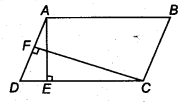Solution:
We know that,
Area of parallelogram = Base x Altitude
Given, AE = 8 cm CF = 10 cm and AB = 16cm
∴ ar (parallelogramABCD) = DC x AE
= 16 x 8 cm2 (∵ AE = 8 cm)…(i)
and ar (parallelogram ABCD) = AD x CF – AD x 10 ( ∵ CF = 10 cm)
From Eq. (i), we have,
16 x 8 = AD x 10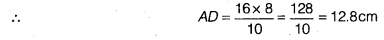Question 2.
If E, F, G and H are respectively the mid-points of the sides of a parallelogram ABCD, show that ar (EFGH) = $$\frac { 1 }{ 2 }$$ ar (ABCD).
Solution:
Given: E,F, G and H are respectively the mid-points of the sides AB, BC, CD and AD. Joint if, it will parallel to CD and AB.
Now, parallelogram HDCF and triangle HGF stand on the same base HF and lie between the same parallel lines DC and HF.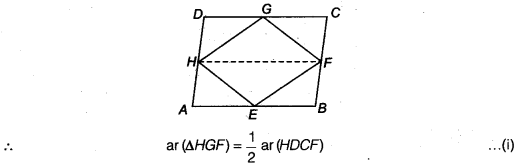Similarly,parallelogram ABFH and triangle HEF stand on the same base HF and lie between the same parallel lines HF and AB.
ar (∆HEF) = $$\frac { 1 }{ 2 }$$ ar (∆BFH) …(ii)
On adding Eqs. (i) and (ii), we get
ar (∆HGF) + ar(∆HEF) = $$\frac { 1 }{ 2 }$$ [ar (HDCF) + ar (ABFH)]
⇒ ar (EFGH) = $$\frac { 1 }{ 2 }$$ ar (ABCD)

Question 3.
P and Q are any two points lying on the sides DC and AD, respectively of a parallelogram ABCD. Show that ar (APB) = ar(BQC).
Solution:
Given: a parallelogram ABCD. P and Q are any two points lying on the sides DC and AD, respectively.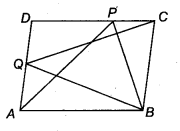Now, parallelogram ABCD and ABQC stand on the same base BC and lie between the same parallel lines BC and AD.
∴ ar (∆ BQC) = $$\frac { 1 }{ 2 }$$ ar(ABCD) …(i)
Similarly, ∆ APB and parallelogram ABCD stand on the same base AB and lie between the same parallels AB and CD.
∴ ar (∆ APB) = $$\frac { 1 }{ 2 }$$ ar (ABCD) ….(ii)
From Eqs. (i) and (ii), we get
ar (∆ APB) = ar (∆ BQC)

Question 4.
In figure, P is a point in the interior of a parallelogram ABCD. Show that
(i) ar (APB) + ar (PCD) = $$\frac { 1 }{ 2 }$$ ar (ABCD)
(ii) ar (APD) + ar(PBC) = ar (APB) + ar (PCD)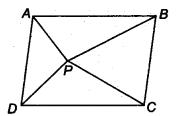Solution:
Given: ABCD is a parallelogram. So, AB || CD, AD |[ BC.
(i) Now, draw MPR parallel to AB and CD both and also draw a perpendicular PS on AB.
∵ MR || AB and AM || BR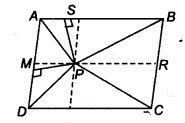∴ ABRM is a parallelogram so
∴ ar (|| gm ABRM) = AB x PS …(i)
and ar (∆ APB) = $$\frac { 1 }{ 2 }$$ x AB x PS
ar (∆ APB) = $$\frac { 1 }{ 2 }$$ ar (|| gm ABRM)
ar (∆PCD) = $$\frac { 1 }{ 2 }$$ ar (|| gm MRCD)
Now, ar (∆APB) + ar (∆ PCD) = $$\frac { 1 }{ 2 }$$ ar (|| gm ABRM) + $$\frac { 1 }{ 2 }$$ ar (||gm MRCD)
= $$\frac { 1 }{ 2 }$$ ar ( || gm ABCD) …(ii)
(ii) Similarly, we can draw a line through P parallel to AD and through the point P draw perpendicular on AD, we cah prove that
ar (∆APD) + ar (∆PBC) = $$\frac { 1 }{ 2 }$$ ar (|| gm ABCD) …(iii)
From Eqs. (ii) and (iii), we get
ar (∆APD) + ar (∆PBQ = ar (∆APB) + ar (∆PCD)
Hence proved.

Question 5.
In figure, PQRS and ABRS are parallelograms and X is any point on side BR. Show that
(i) ar (PQRS) = ar (ABRS)
(ii) ar (AXS) = $$\frac { 1 }{ 2 }$$ ar (PQRS)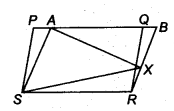Solution:
Given: PQRS and ABRS both are parallelogram and x is any point on BR.
(i) Here, parallelogram PQRS and ABRS lies on the same base SR and between the same parallel lines SR and PB.
∴ ar (PQRS) = ar (ABRS) …(i)
(ii) Again, in parallelogram ABRS, ∆ AXS and parallelogram lies on the same base AS and between the same parallel lines AS and BR.
∴ ar (∆AXS) = $$\frac { 1 }{ 2 }$$ar (∆BRS) …(ii)
Now, from Eqs. (i) and (ii), we get
ar (∆AXS) = $$\frac { 1 }{ 2 }$$ ar (∆ PQRS)

Question 6.
A farmer was having a field in the form of a parallelogram PQRS. She took any point A on RS and joined it to points P and Q. In how many parts the fields is divided? What are the shapes of these parts? The farmer wants to sow wheat and pulses in equal portions of the field separately. How should she do it.
Solution:
Given: PQRS is a parallelogram and A is any point as RS. Now, join PA and PQ. Thus, the field will be divided into three parts and each part is in the shape of a triangle.
Since, the AAPQ and parallelogram PQRS lie on the same base PQ and between same parallel lines PQ and SR.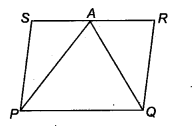∴ ar (∆APQ) = $$\frac { 1 }{ 2 }$$ ar (PQRS) ….(i)
Then, remaining
∴ ar (∆ASP) + ar (∆ARQ) = $$\frac { 1 }{ 2 }$$ ar (PQRS) ….(ii)
Now, from Eqs. (i) and (ii), we get
ar (∆APQ) = ar (∆ASP) + ar (∆ARQ)
So, farmer has two options.
Either the farmer should sow wheat and pulses in ∆APS and ∆AQR or in ar [∆APQ and (∆APS and ∆AQR)] separately.
We hope the NCERT Solutions for Class 9 Maths Chapter 10 Areas of Parallelograms and Triangles Ex 10.2 help you. If you have any query regarding NCERT Solutions for Class 9 Maths Chapter 10 Areas of Parallelograms and Triangles Ex 10.2, drop a comment below and we will get back to you at the earliest.